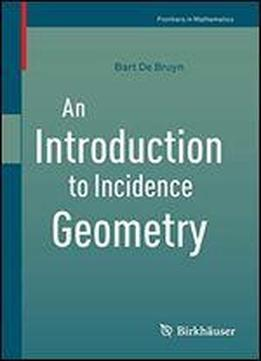# An Introduction To Incidence Geometry (frontiers In Mathematics) by Bart De Bruyn / 2016 / English / PDF

This book gives an introduction to the field of Incidence Geometry by discussing the basic families of point-line geometries and introducing some of the mathematical techniques that are essential for their study. The families of geometries covered in this book include among others the generalized polygons, near polygons, polar spaces, dual polar spaces and designs. Also the various relationships between these geometries are investigated. Ovals and ovoids of projective spaces are studied and some applications to particular geometries will be given. A separate chapter introduces the necessary mathematical tools and techniques from graph theory. This chapter itself can be regarded as a self-contained introduction to strongly regular and distance-regular graphs. This book is essentially self-contained, only assuming the knowledge of basic notions from (linear) algebra and projective and affine geometry. Almost all theorems are accompanied with proofs and a list of exercises with full solutions is given at the end of the book. This book is aimed at graduate students and researchers in the fields of combinatorics and incidence geometry.

views: 384